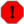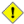## Name

= — Returns `TRUE` if A's bounding box is the same as B's. Uses double precision bounding box.

## Synopsis

`boolean =(` geometry A , geometry B `)`;

`boolean =(` geography A , geography B `)`;

## Description

The `=` operator returns `TRUE` if the bounding box of geometry/geography A is the same as the bounding box of geometry/geography B. PostgreSQL uses the =, <, and > operators defined for geometries to perform internal orderings and comparison of geometries (ie. in a GROUP BY or ORDER BY clause).This is cause for a lot of confusion. When you compare geometryA = geometryB it will return true even when the geometries are clearly different IF their bounding boxes are the same. To check for true equality use ST_OrderingEquals or ST_EqualsThis operand will NOT make use of any indexes that may be available on the geometries.This method supports Circular Strings and CurvesThis function supports Polyhedral surfaces.

Changed: 2.0.0 , the bounding box of geometries was changed to use double precision instead of float4 precision of prior. The side effect of this is that in particular points in prior versions that were a little different may have returned true in prior versions and false in 2.0+ since their float4 boxes would be the same but there float8 (double precision), would be different.

## Examples

```SELECT 'LINESTRING(0 0, 0 1, 1 0)'::geometry = 'LINESTRING(1 1, 0 0)'::geometry;
?column?
----------
t
(1 row)

SELECT ST_AsText(column1)
FROM ( VALUES
('LINESTRING(0 0, 1 1)'::geometry),
('LINESTRING(1 1, 0 0)'::geometry)) AS foo;
st_astext
---------------------
LINESTRING(0 0,1 1)
LINESTRING(1 1,0 0)
(2 rows)

-- Note: the GROUP BY uses the "=" to compare for geometry equivalency.
SELECT ST_AsText(column1)
FROM ( VALUES
('LINESTRING(0 0, 1 1)'::geometry),
('LINESTRING(1 1, 0 0)'::geometry)) AS foo
GROUP BY column1;
st_astext
---------------------
LINESTRING(0 0,1 1)
(1 row)

-- In versions prior to 2.0, this used to return true --
SELECT ST_GeomFromText('POINT(1707296.37 4820536.77)') =
ST_GeomFromText('POINT(1707296.27 4820536.87)') As pt_intersect;

--pt_intersect --
f
```